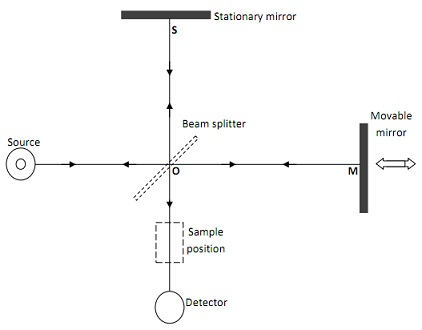#### Fourier Transform Spectroscopy, Chemistry tutorial

Introduction:

Fourier transform spectroscopy is a non dispersive technique that utilizes an array of detectors to calculate the complete spectrum at once or all together. The spectrum is swelling into its individual component wavelengths and each small band of wavelength is directed onto one detector. Therefore, Fourier analysis is a mathematical technique to decompose a signal into its component wavelengths that can be computed at once.

Definition:

The Fourier transform infrared (FTIR) instruments don't enclose any dispersing device and all wavelengths are detected and calculated simultaneously. In this case, in its place of monochromator, a device termed interferometer is utilized to generate interference patterns that enclose the infrared spectral information. Therefore, the technique is termed interferometry.

Interferometer:

Interferometer is a non-dispersive machine that attains spectral information through constructive and destructive interference utilized in Fourier Transform Infrared instrument.

An interferometer is the heart of a FTIR spectrophotometer. It consists of a collimated light source, a stationary mirror at the top, a variable mirror at the right, a beam splitter and a detector. The light source might be a laser or sodium arc lamp. The mirrors are accuracy polished ultra-flat glass by a reflecting coating vapour deposited on their surface. The movable mirror is mounted on an extremely accurate linear bearing, which permits it to move along the direction of the light beam while continuing perpendicular to it.

The beam splitter is the key heart to the operation of the interferometer. It permits a fraction of the light to pass through the mirror and reflect the other fraction or part. The machine efforts in both directions so that light falling on either side of the beam splitter is incompletely reflected and partially transmitted.

Instrumentation:

The instrumental demonstration of an interferometer is revealed below:Fig: A schematic diagram of interferometer

As seen in the above diagram, a beam from the light source falls on the splitter which then splits the beam into two parts. The two beams travel in separate paths and converge on the detector. The two beams (OS and OM) converge in the same region of space and form an interference pattern. As the movable mirror in the right is moved, the interference pattern shifts across the detector and modulates the optical signal. The resulting reference interferogram which is a plot of output light intensity against the wave number (1/λ) is recorded. This is used as a measure of the power of the incident beam at all wavelengths. An absorbing sample is then inserted into the beam, and a sample interferogram is recorded. The two interferograms are then used to compute the absorption spectrum of the sample.Fig: an interferogram of the light source

Sample application

In a Fourier spectrophotometer, the example in a cell is generally situated between the output of the interferometer and the detector. As the light is passing through the model, it then absorbs assured wavelengths of the incident light. Because the sample absorbs definite wavelengths of light, the interferogram encloses the spectrum of the source minus the spectrum of the example. An interferogram of a reference sample (blank) enclosing only the solvent is 1st recorded and transformed into a example spectrum. The 2 interferograms are then utilized to calculate the absorption spectrum of the example.

The FTIR spectrophotometer is a extremely sophisticated instrument. It has benefits of: Speed, frequency accuracy, more competent utilize of radiation via the interferometer, improved signal, a built data handling capabilities. Such benefits reason the quick displacement of traditional dispersion instruments.

Tutorsglobe: A way to secure high grade in your curriculum (Online Tutoring)